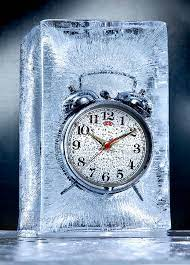## How to Calculate and Solve for Freezing Time | Solidification of MetalsThe image above represents freezing time.

To compute for freezing time, three essential parameters are needed and these parameters are Thickness of Solidified Metal (M), Constant (β) and Heat Diffusivity (α).

The formula for calculating freezing time:

t = / 4β²α

Where:

t = Freezing Time
M = Thickness of Solidified Metal
β = Constant
α = Heat Diffusivity

Let’s solve an example;
Find the freezing time when the thickness of solidified metal is 8, the constant is 10 and the heat diffusivity is 2.

This implies that;

M = Thickness of Solidified Metal = 8
β = Constant = 10
α = Heat Diffusivity = 2

t = / 4β²α
t = / 4(10)²(2)
t = 64 / 4(100)(2)
t = 64 / 800
t = 0.08

Therefore, the freezing time is 0.08 s.

Calculating the Thickness of Solidified Metal when the Freezing Time, the Constant and the Heat Diffusivity is Given.

M = t x 4β²α

Where:

M = Thickness of Solidified Metal
t = Freezing Time
β = Constant
α = Heat Diffusivity

Let’s solve an example;
Find the thickness of solidified metal when the freezing time is 21, the constant is 9 and the heat diffusivity is 2.

This implies that;

t = Freezing Time = 21
β = Constant = 9
α = Heat Diffusivity = 2

M = t x 4β²α
M = 21 x 4(9²)(2)
M = 21 x 4(81)(2)
M = 21 x 4(162)
M = 21 x 648
M = 13668

Therefore, the thickness of solidified metal is 13668.